﻿ Possibilities of Optimizing the Shape and Geometry of Castellated Beams Using Numerical and Experimental Modeling

### Possibilities of Optimizing the Shape and Geometry of Castellated Beams Using Numerical and Experime...

Miroslav Pástor, Jozef Bocko, Tomáš Kula, Cosmin-Stefan Roszkos

American Journal of Mechanical Engineering

## Possibilities of Optimizing the Shape and Geometry of Castellated Beams Using Numerical and Experimental Modeling

Miroslav Pástor1, Jozef Bocko1, Tomáš Kula1,, Cosmin-Stefan Roszkos1

1Department of Applied Mechanics and Mechanical Engineering, Technical University of Košice, Faculty of Mechanical Engineering, Letná 9, Košice, 04200, Slovakia

### Abstract

The article discusses about numerical methods and physics experiment for studying stress fields caused by defined load on beam. Examined the behavior of material under various modes load at individual types of beams. The results are compared, and then the right conclusions are drawn, that it is possible to verify the physical experiment and numerical calculation. For physical experiment was used transmission photoelasticimetry.

• Miroslav Pástor, Jozef Bocko, Tomáš Kula, Cosmin-Stefan Roszkos. Possibilities of Optimizing the Shape and Geometry of Castellated Beams Using Numerical and Experimental Modeling. American Journal of Mechanical Engineering. Vol. 4, No. 7, 2016, pp 357-362. http://pubs.sciepub.com/ajme/4/7/22
• Pástor, Miroslav, et al. "Possibilities of Optimizing the Shape and Geometry of Castellated Beams Using Numerical and Experimental Modeling." American Journal of Mechanical Engineering 4.7 (2016): 357-362.
• Pástor, M. , Bocko, J. , Kula, T. , & Roszkos, C. (2016). Possibilities of Optimizing the Shape and Geometry of Castellated Beams Using Numerical and Experimental Modeling. American Journal of Mechanical Engineering, 4(7), 357-362.
• Pástor, Miroslav, Jozef Bocko, Tomáš Kula, and Cosmin-Stefan Roszkos. "Possibilities of Optimizing the Shape and Geometry of Castellated Beams Using Numerical and Experimental Modeling." American Journal of Mechanical Engineering 4, no. 7 (2016): 357-362.

 Import into BibTeX Import into EndNote Import into RefMan Import into RefWorks

### 1. Introduction

Start of development of thin-walled structures can be traced back to the 19th century, due to the rapid development of the industry, it was necessary to create large-scale buildings, structures and facilities, when conventional design methodologies together with traditional materials could not be always applied. The term thin-walled elements we can imagined from the simple, such as is steel wall panels to the various shell sections, and the rings with a defined wall thickness, which is relative to the other dimensions significantly smaller .

Castellated beams are known as an effective alternative to standard profiles. They are made from rolled and welded sections, when the web is cutting on two symmetric sides and subsequent is welded. Following such an adjustment of beam it is increasing its height with created holes in the middle of the web, which contributes to the bending stiffness while maintaining low weight. Course there is fact, that such structural update is positively reflected in the final price of the beam. Castellated beams have application in various technical works for example roof constructions (Figure 1), the structure for the crane line, wherever, where are the emphasis on the maximum capacity with minimum weight.Download asVeiw figureFigures index
Figure 1. View of the roof structure formed from the castellated beams 

Another advantage is the possibility of castellated beams crossing installation distributions through web and connection with concrete and thus, creates optimal joint connection and the possibility of manufacturing complex shaped or curved shapes according to drawing specifications.

Standardly produced types of beams represent the following groups:

a) Castellated symmetrical beam with a hexagonal hole (Figure 2a). This base type beam predominantly used as a bending stress component of steel structures.

b) Castellated symmetrical beam with a circular hole (Figure 2b). This type of beam is particularly suitable for uniform load because it has less resistance for shear loading.

c) Castellated asymmetric beam with a hexagonal hole (Figure 2c). This type of beam has application in cases, where the stress of the two flanges is different and not symmetrical. This is the case for example of composite steel structures that are not protected against lateral buckling .Download asVeiw figureFigures index
Figure 2. Typology of castellated and cellular beams

It should be noted, that in place with high level of displacing forces are holes welded with additional material or is necessary use fixing ribs.

### 2. Stress Analysis of Castellate Beam Using Numerical and Experimental Modeling

The article is about a methodology for stress analysis of beam with holes, using optical methods and numerical modeling by finite element method (FEM).

Considering that the goal was to perform stress analysis around concentrators (holes), was selected for the stress analysis as vertical part of the beam - the web. The paper describes approach of stress analysis of castellated beam comprising from two parts at different modes of loading. The main objective was to assess the impact of links both parts of the beam on the distribution of stress field. Analyzed a simplified model of the beam consists of:

1. fixed linked parts,

2. not connected parts.

Realm load forces are chosen so as to create:

Description of Reference analyzed variants are listed in Table 1.

#### Table 1. Description of analyzed variants

2.1. Making – CAD and FEM Models
2.1.1. CAD Beam Model with Holes

CAD model was created in an environment of Siemens NX 10. Analyzed model is based on the real master, where castellated beams are used for construction of the supporting bridge construction (Figure 3).Download asVeiw figureFigures index
Figure 3. Bridge construction created by castellated beams 

In the production of beam model with defined holes (Figure 4), has been applied law of model similarity in order to use the physical model for the experimental measurements.Download asVeiw figureFigures index
Figure 4. CAD beam model with holes

During development, the authors had to consider size of the sensitive plate, which was used for the production of a physical model. External dimensions of the model are length 500 mm, height 60 mm and width 6,25 mm. In Figure 5 show a detail of the shape hole and dimensions.Download asVeiw figureFigures index
Figure 5. Shape and dimensions of hole

2.1.2. FEM Model

Nowadays, the finite element method (FEM) is the most popular and spread method of computation in continuum mechanics [4, 5]. A deformation variant has been expanded in practice. From numerical point of view it is numerical method of approximation of boundary problem. The body (Figure 6) is replaced by union of set of sub regions, which we call finite elements.Download asVeiw figureFigures index
Figure 6. Solution of boundary problem

The finite element method can be based e.g on the principle virtual displacements. At the element level we can write equation: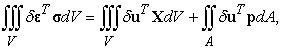(1)

where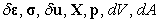is variation of strain vector, stress vector, variation of displacement vector, body force vector, pressure vector, infinitesimal volume and infinitesimal area, respectively. Displacement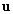can be expressed as: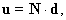(2)

where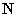is matrix containing the shape functions and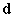is vector of node displacement.

Now we have equation: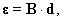(3)

which expresses the dependence of the strain vector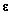on the node displacement vectorand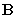is matrix containing derivatives of shape functions.

In case of linear elastic material we have relation: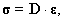(4)

where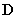is matrix of elastic constants. Further we use equation (3) and we get: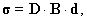(5)

and final relation: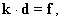(6)

where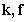is element stiffness matrix and nodal load vector, respectively. For the whole body we have equation: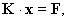(7)

where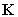is global stiffness matrix,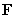is resultant vector of load forces and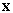is displacement vector of the whole structure .

The model of beam is formed with the finite element mesh what is shown in Figure 7a. Detail view of soften mesh around concentrators is shown in Figure 7b. Boundary conditions for the different variants are shown in Figure 8a (symmetrical load), Figure 8b (asymmetrical load).Download asVeiw figureFigures index
Figure 7. Mesh of finite elements a) whole model, b) detail of mesh around concentratorsDownload asVeiw figureFigures index
Figure 8. Defined boundary conditions for load condition a) Variant A, C, b) Variant B, D

Calculation parameters are:

- the type of analysis: SOL 101 Linear Statics - Global Constraints,

- type of finite element: CTETRA (10),

- material: PSM-1,

Because experimental measurements will be made for full-field stress analysis of equal difference of principal stresses, in Figure 9 until Figure 12 are shown details of equal difference of principal stresses σ1 - σ3 around the concentrator for each variant.Download asVeiw figureFigures index
Figure 9. Field of equal difference of principal stresses σ1 - σ3 around the concentrator – variant ADownload asVeiw figureFigures index
Figure 10. Field of equal difference of principal stresses σ1 - σ3 around the concentrator – variant BDownload asVeiw figureFigures index
Figure 11. Field of equal difference of principal stresses σ1 - σ3 around the concentrator – variant CDownload asVeiw figureFigures index
Figure 12. Field of equal difference of principal stresses σ1 - σ3 around the concentrator – variant D

Based on the full-field stress analysis, it could be stated, that in the unconnected parts of the model, there is a signigicant change in the stress field distribution at the top and the bottom of castellated beam, compared with the model which is made with fixed connection. The values of the stress intensity are shown in Table 2.

#### Table 2. Maximum values of stress intensity

The accuracy of obtained results was, verified by experimental measurements on a physical model made of optically sensitive material.

2.2. Experimental Stress Analysis

Experiment fulfill in the design of technical objects with different functions, which can be summarized as follows :

- input experiment is often the only means, to solve the problem where, there is no theory to solve the problem or not feasible respectively. Experimental approach is more effective than computational approach,

- formulation experiment it is a means, to verify the truthfulness of the theory,

- verification experiment it is a means, for confrontation and verification of results obtained by calculating with experimental results,

- output experiment based on the experimental evidence, is possible to create simpler computational and experimental models,

- concretized experiment it is a means, to obtain input data for calculation algorithms, both of straight and inverse problems,

- identification experiment it is a means, to identify the structure of objects.

Experiment does not fulfill an important role only at the design stage of the individual components and the entire technical object, but also in the final stages. In this paper is used methodology of verification experiment, where the results of the physical model are compared with results of numerical modeling.

The transmission photoelasticimetry is an experimental method enabling stress analysis within the investigation of a stress field [7, 8]. It is the ability for immediate identification of the nominal amounts of stress, stress gradient and the total stress distribution - including identification of congested and less loaded areas . This feature is described as full-field interpretation and is unique in the methods of photoelasticity and stress analysis. Its successful application depends on the recognition order isochromatic lines by color and by understanding the relationship between the order of the fringe and the value of the stress .

Overall photoelastic patterns usually indicate corrective measures for the prevention of breach often based thereon leads to removal of material and thus weight reduction of examined objects.

For full-field stress analysis was used polariscope - Model 060 from Measurements Group, Inc. It is a precision optical device for the quantitative determination of stress and consists of polarization and analysis system, and a white light source. During stress detection on analyzed samples, photoelastic model is placed in a workplace of polariscope. With gradual load model, generated color lines in places with high levels of stress and spread to the areas with low levels of stress. Isochromatic lines are the geometric trace of equal difference of principal stresses σ1 - σ3. The article describes a method of full-field stress analysis based on recognition of the order by isochromatic color lines.

For experimental measurement was made model of a castellated beam from optically sensitive material PSM-1 of one part (Figure 13a) and consisting of two separate parts (Figure 13b). For elimination of residual stresses, was for model production used water jet cutting technology. The properties of the used material PSM-1 are present in Table 3.Download asVeiw figureFigures index
Figure 13. The models of the castellated beam produced from optically sensitive material PSM-1

At Figure 14 is a measuring stand in the workspace of polariscope. Beam loading was caused by gravity rod stored in roller bearing at the specified location.

#### Table 3. Properties of optically sensitive material PSM-1

At Figure 15 is view at models without loads. Experimental full-field stress analysis was carried under conditions corresponding to boundary conditions defined in numerical modeling.Download asVeiw figureFigures index
Figure 14. Measuring stand in the polariscope workspaceDownload asVeiw figureFigures index
Figure 15. Castellated beams without loads a) connected parts, b) not connected parts

In Figure 15b is documented rise isochromatic lines in weakened cross section, which in the case of the beam consisting of two unconnected parts can be regarded as self-weight impact.

In Figure 16 to Figure 19 are documented fields of isochromatics around the concentrator for variants A - D.Download asVeiw figureFigures index
Figure 16. Field of isochromatics around analyzed concentrators – variant ADownload asVeiw figureFigures index
Figure 17. Field of isochromatics around analyzed concentrators – variant BDownload asVeiw figureFigures index
Figure 18. Field of isochromatics around analyzed concentrators – variant CDownload asVeiw figureFigures index
Figure 19. Field of isochromatics around analyzed concentrators – variant D

Preferably selected optical method is relatively quick identification of locations with high stress gradient. Areas with high gradient of stress are characterized by a high density of isochromatics.

At Figure 16 and Figure 17 are isochromatic lines at the model with symmetrical and asymmetrical load. The above figure shows that under specified load has no increased significantly stress gradient. In Figure 18 and Figure 19 are documented isochromatics for the same load on the model made with two no connected parts. The pictures presented significant increase isochromatic lines representing an increase stress levels in the analyzed areas. Based on a comparison of field of isochromatics on both models, it can be concluded, that increased levels of stress was due to the different parts of the deposit analyzed castellated beam.

Methods of experimental measurements may e.g. analyzed different combinations of deposit (connection) weakened parts in selected locations, etc..

Based on numerical and experimental data, it can be say that is good result agreement.

Although the castellated beams are widely used particularly in the design of load-bearing parts of the roof structure itself it is further to optimize the shape and geometry of the beams also performs buckling strength and stiffness control. Failure to properly design of the shape or geometry may be permanent damage to such proposed beams (Figure 20).Download asVeiw figureFigures index
Figure 20. Collapsed cellular beam 

### 3. Conclusion

Numerical modeling is currently the most widely used tool in the design of load-bearing elements, machinery and equipment, etc. It should be noted that the accuracy of the results of numerical modeling depends mainly on defining the correct boundary conditions, selected the type of finite element and mesh density, etc. The paper describes the procedure of verification accuracy of the results obtained by numerical modeling. Based on an analysis of the beam results with hexagonal holes, using numerical modeling and experimental measurements using transmission photoelasticimetry can be stated accuracy of the proposed methodology. The advantage of the experimental measurements in addition to the possibility to repeat measurements (in the material elastic area) is also relatively quickly assess the impact of different modes of load or deposit beam on full-field stress analysis. Experimental Stress analysis using the optical methods can be a useful instrument for optimization the shape and geometry of the holes and distance between them to reduce the levels of stress in the critical areas. According to the law of model similarity, experimental measurements can be also transforming the real structure.

### Acknowledgements

This paper was supported by project by projects VEGA No. 1/0751/16 and VEGA No. 1/0731/16.

### References

  Novotný, T. and Otisk, D. Prelamované nosníky směrnica 11. [E-book]Available:http://www.noving.cz/web/_docs/smernice/Prolamovaky_Smernice.pdf.In article  http://www.grayson-engineering.co.nz/capability.php?a=CellularBeamsIn article  http://www.cellularbeam.net/productlist.php.In article  Zienkiewicz, O.C., Taylor, R.L., Zhu, J.Z., The Finite Element Method: Its Basis and Fundamentals, Butterworth-Heinemann, United Kingdom, 2013.In article  Reddy, J.N., An Introduction to the Finite Element Method, McGraw-Hill, 2005, pp. 912.In article  Trebuňa, F. and Šimčák, F., Handbook of Experimental Mechanics (in Slovak), Typopress, Košice, 2007.In article  Laermann, K. H., Optical Methods in Experimental Solid Mechanics. Springer Wien New York 2000.In article View Article  Patterson, A. E. Digital Photoelasticity: Principles, Practice and Potential. In: Strain, Volume 38, Issue 1, February 2002, Pages: 27-39.In article View Article  Pástor, M. and Trebuňa, F. Application of transmission photoelasticimetry for stress concentration analyses in construction supporting parts. Applied Mechanics and Materials 611, pp. 443-449 (2014).In article View Article  Ramesh, K., Digital Photoelasticity – Advanced Techniques and Applications, Springer -Verlag, Berlin, Germany, 2000.In article View Article  Durif, S. - Bouchaïr, A. - Vassart, O. Validation of an analytical model for curved and tapered cellular beams at normal and fire conditions. Civil Engineering 57 / 1 (2013) 83-95.In article